# GMAT Math : DSQ: Calculating the area of an equilateral triangle

## Example Questions

2 Next →

### Example Question #431 : Data Sufficiency Questions

Given two equilateral triangles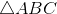and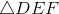, which has the greater area?

Statement 1: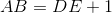Statement 2: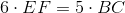Statement 2 ALONE is sufficient to answer the question, but Statement 1 ALONE is NOT sufficient to answer the question.

EITHER statement ALONE is sufficient to answer the question.

BOTH statements TOGETHER are sufficient to answer the question, but NEITHER statement ALONE is sufficient to answer the question.

Statement 1 ALONE is sufficient to answer the question, but Statement 2 ALONE is NOT sufficient to answer the question.

BOTH statements TOGETHER are insufficient to answer the question.

EITHER statement ALONE is sufficient to answer the question.

Explanation:

Since the area of an equilateral triangle is solely dependent on the length of one side, it follows that the triangle with the greater sidelength has the greater area.

Statement 1 alone gives that the length of one side ofis greater than that on one side of.It follows thathas the greater area.

Statement 2 alone gives that, from which it follows that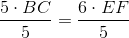and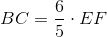.

Again, this shows thathas the greater sidelength and the greater area.

### Example Question #72 : Triangles

Give the area of equilateral triangle.

Statement 1: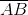is a diameter of a circle with circumference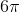.

Statement 2: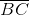is a side of a 45-45-90 triangle with area.

Statement 1 ALONE is sufficient to answer the question, but Statement 2 ALONE is NOT sufficient to answer the question.

Statement 2 ALONE is sufficient to answer the question, but Statement 1 ALONE is NOT sufficient to answer the question.

EITHER statement ALONE is sufficient to answer the question.

BOTH statements TOGETHER are insufficient to answer the question.

BOTH statements TOGETHER are sufficient to answer the question, but NEITHER statement ALONE is sufficient to answer the question.

Statement 1 ALONE is sufficient to answer the question, but Statement 2 ALONE is NOT sufficient to answer the question.

Explanation:

Assume Statement 1 alone. To find the diameter of a circle with circumference, divide the circumference byto get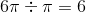. This is also the length of each side of the triangle, so we can get the area using the area formula: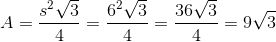.

Assume Statement 2 alone. A 45-45-90 Triangle has congruent legs, and the area is half the product of their lengths, so if we letbe the common sidelength,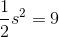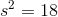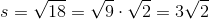By the 45-45-90 Theorem, the hypotenuse has lengthtimes this, or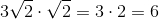.

Since it is not given whetheris a leg or the hypotenuse of a right triangle, however, the length of- and consequently, the area - is not clear.

### Example Question #71 : Triangles

Find the area of an equilateral triangle.

1. A side measures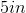.
2. An angle measures.

Statements 1 and 2 are not sufficient, and additional data is needed to answer the question.

Statement 1 alone is sufficient, but statement 2 alone is not sufficient to answer the question.

Statement 2 alone is sufficient, but statement 1 alone is not sufficient to answer the question.

Both statements taken together are sufficient to answer the question, but neither statement alone is sufficient.

Each statement alone is sufficient to answer the question.

Statement 1 alone is sufficient, but statement 2 alone is not sufficient to answer the question.

Explanation:

In an equilateral triangle all sides are of the same length and all internal angles measure toStatement 1: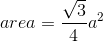Whererepresents the length of the side.

If we're given the side, we can calculate the area: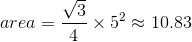Statement 2: We don't need the angle to find the area.

2 Next →

### All GMAT Math Resources